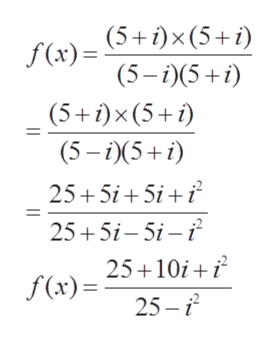Write the quotient in standard form: f (x) = 5+i / 5−i

Question

Write the quotient in standard form: f (x) = 5+i / 5−i

Step 1

we are given a complex value as

Step 2

For simplifying it , we can rationalize it

and for rationalizing it , we can multiply conjugate of denominator on both top and bottom

Step 3

We can distribute and then we can si...help_outlineImage Transcriptionclosef (x)= (5+1)x(5+i (5-i)(5 (5 )x(5 (5-i)5+i) 25+5i+5i 2 25 5i-5i i f(x)= 2510i+i 25-i fullscreen

Want to see the full answer?

See Solution

Want to see this answer and more?

Our solutions are written by experts, many with advanced degrees, and available 24/7

See Solution
Tagged in

Complex Numbers